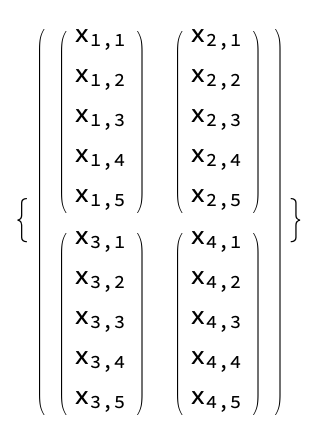# Speeding up custom convolutions for multiple CPUS

Hi I’m trying to implement a convolution that operates on a slighly different image representation.

This representation can be though of as taking an 2D image with pixel values `x_i`I see that this feels similar as a multi channel image, but the import difference is the inner most vectors should be treated as a single object and added element wise. For example a more complex image like

When convolved with the kernelWould output this mess

The other fun twist is bias must only be added to the first element of every pixel vector.

I have a simple implementation ignoring strides and padding that looks like this

``````    def forward(self, img):
k = self.kernel_size
# dimension of each image channel (assumes n x n)
n = img.shape[-2]

# <5> stack all output channels
# <4> take all channels, stack and sum
[torch.stack(
# <3> takes all rows and stack
[torch.stack(
# <2> take inner products across a row and stack
[torch.stack(
# <1> take inner product with kernel at top left corner pixel (l, m)
[torch.stack([kernel[i, j] * img_channel[l + i, m + j] for i in range(k) for j in
# <1>
for m in range(n - k)])
# <2>
for l in range(n - k)])
# <3>
for img_channel in img]).sum(0)
# <4>
for kernel, bias in zip(self.weight, self.bias)])
# <5>
``````

I figured since this is really so similar to regular convolution exept that pixels are now vectors and added elementwise someone might have an idea for how I could use some pytorch builtins more effectively. Since I will be running this on a 14 core machine this nasty thing of list comprehensions will really hurt my performance.

Any ideas are much appreciated. Thanks

Hi,

I can take a stab at this.
Could you make a self contained script with your implementation, random inputs of the exact shape you’re interested in (including batch potentially and specifying which dimension is which with your math above) and the expected output?

Sure just give me a minute.

Here’s a self contained script with a short description and several test cases. The help is really appreciated. Thx

Hey,

Here is an implementation that should be significantly faster and match what you want. Because of the very different low precision of your ground truth, the test trips, but comparing the two forward passes lead to very small error (same up to 5 places):

``````class Conv2D(torch.nn.Module):
def __init__(self, in_channels, out_channels, kernel_size, stride=1, padding=0):
super(Conv2D, self).__init__()
# These are ones only for testing
self.weight = Parameter(torch.ones(out_channels, in_channels, kernel_size, kernel_size), requires_grad=False)
self.kernel_size = kernel_size
self.stride = stride

def forward(self, img):
# img is (num_channels x n x n x num_errors)
# outer dimension of each image channel (assumes n x n)
num_channels = img.size(0)
n = img.shape[-2]
# kernel is square
k = self.kernel_size
# padding is the same in both H and W
Note that you could do similar stuff with a batch of input by doing a similar permute as `(0, -1, 1, 2, 3)`. Then collapse the first dimension in a common batch. After the conv, uncollapse the two dimensions and permute back.